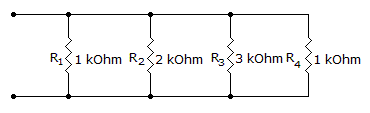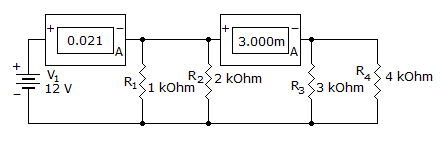# Electronics - Parallel Circuits

Exercise : Parallel Circuits - General Questions
21.
When a parallel 5 kresistor and a 25 kresistor have a 10 V supply, what is the total power loss?
2.4 mW
3.3 mW
24 mW
33 mW
Explanation:
No answer description is available. Let's discuss.

22.
In a parallel resistive circuit:
there is more than one current path between two points
the voltage applied divides between the branches
the total branch power exceeds the source power
the total circuit conductance is less than the smallest branch conductance
Explanation:
No answer description is available. Let's discuss.

23.
What is the total resistance?7 k1 k706353Explanation:
No answer description is available. Let's discuss.

24.
Kirchhoff's current law for parallel circuits states that the:
sum of all branch voltages equals zero
total circuit resistance is less than the smallest branch resistor
sum of currents into a junction is equal to the difference of all the branch currents
sum of the total currents flowing out of a junction equals the sum of the total currents flowing into that junction
Explanation:
No answer description is available. Let's discuss.

25.
Which component is open?R1
R2
R3
R4